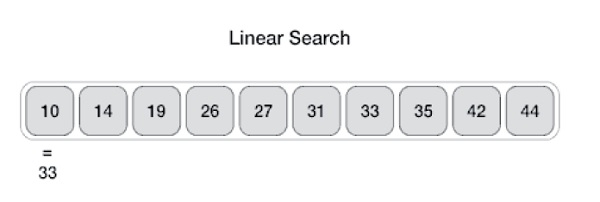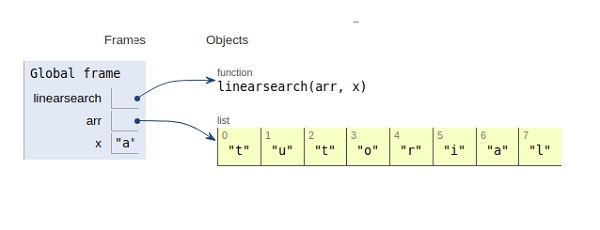# Python Program for Linear Search

In this article, we will learn about the Linear Search and its implementation in Python 3.x. Or earlier.

## Algorithm

Start from the leftmost element of given arr[] and one by one compare element x with each element of arr[]
If x matches with any of the element, return the index value.
If x doesn’t match with any of elements in arr[] , return -1 or element not found.

Now let’s see the visual representation of the given approach −## Example

Live Demo

def linearsearch(arr, x):
for i in range(len(arr)):
if arr[i] == x:
return i
return -1
arr = ['t','u','t','o','r','i','a','l']
x = 'a'
print("element found at index "+str(linearsearch(arr,x)))

## Output

element found at index 6

The scope of the variables are shown in the figure −